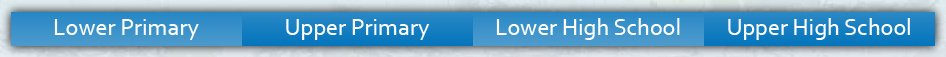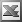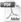Lower High School Enter keywords to search by topic or worksheet description

Worksheets in this category assist provide students with an understanding of analytical geometry through exercises involving linear and quadratic functions,

Worksheet Name Files
Linear Equations: Plotting Points & GraphingGradient of a Line SegmentFinding Gradient Given Two PointsLinear Equations: Matching Equations with GraphsLinear Equations: Finding the Equation from a GraphEquation to a Line: Given Slope & One PointEquation to a Line: Given Two PointsEquations of Parallel LinesEquations of Perpendicular LinesMidpoint of a Line SegmentDistance Between Two PointsQuadratic Equations: Plotting Points & GraphingQuadratic Equations: Curve Sketching